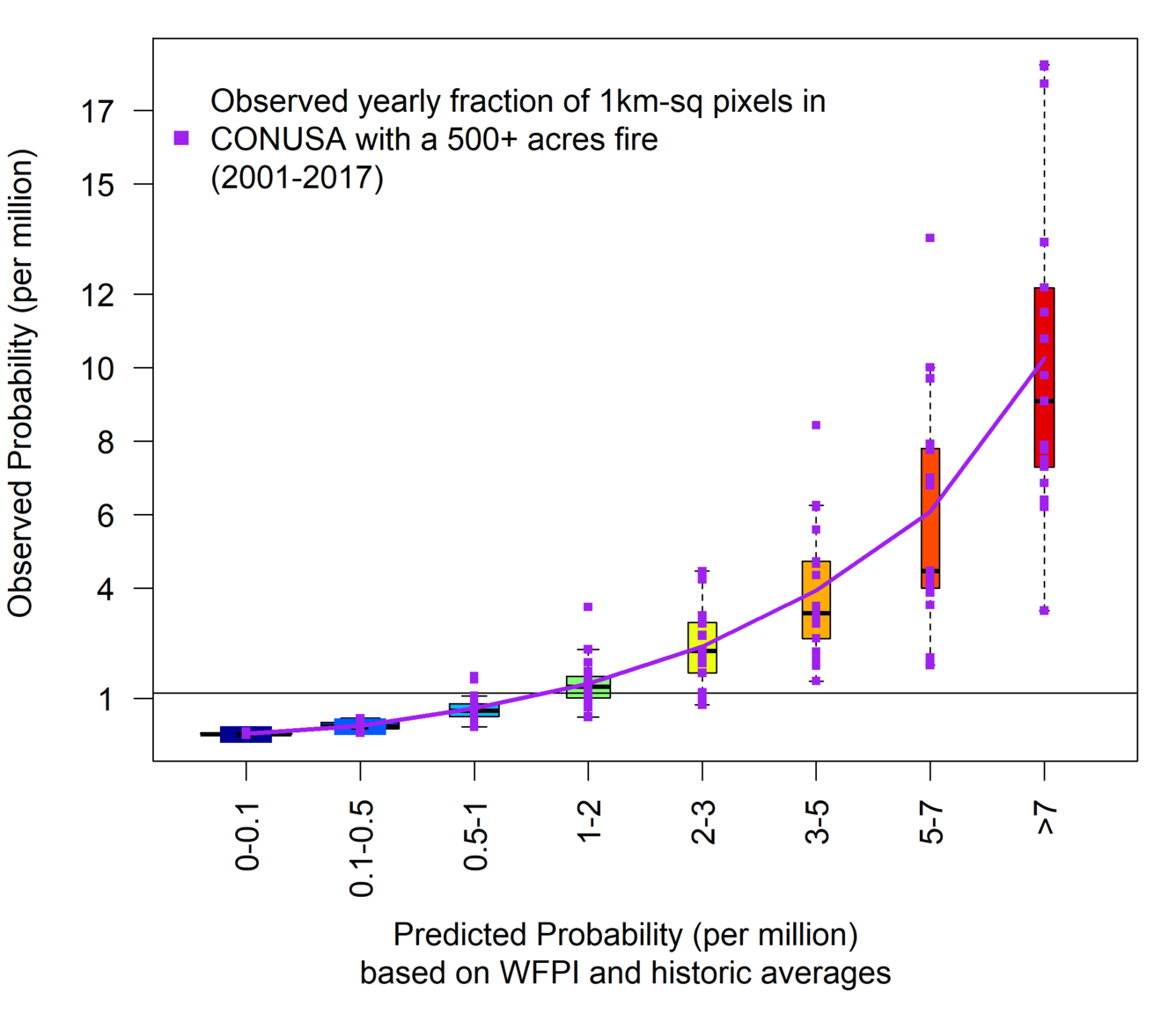An official website of the United States government

# WFPI-based Large Fire Probability (WLFP)

WLFP (Figure 1) is an estimate of risk (probability) that a new fire will burn to more than 500 acres (202 ha).

Large fire probability predictions are based on the historic average probability of a large fire adjusted, up or down, by the WFPI value for that day. Historic averages are specific for each location and day-in-year. Fire occurrence data from the period 2001-2017 were used to evaluate average probabilities and WFPI adjustments.

Historically, higher numbers of large fires (>500 acres) have occurred at the highest levels of WLFP (Table 1).

Table 1: Observed proportion of large fires (>500 acres) per voxel (km2-day) at each predicted WLFP group level.

Predicted WLFP

(per million voxels)

Observed Proportion1

(per million voxels)

0.0 – 0.1 0.04
0.1 – 0.5

0.26

0.5 – 1.0       0.72
1.0 – 2.0 1.38
2.0 – 3.0 2.40
3.0 – 5.0 3.88
5.0 – 7.0 5.57
> 7.0 9.78

1 Observed proportion based on the historic period 2001-2017

The accuracy of the predictions is demonstrated by the reliability diagram (Figure 2.), where observed values are plotted against predicted values.  Here, observed values were evaluated by counting the fraction of voxels, in the contiguous US per year, with 500+ fires in each of the eight WLFP category. Calculations of observed fractions were done for each year (2001-2017) separately using cross validation. Specifically, the predicted probabilities for each voxel were evaluated using data from all other years excluding the year in question.Figure 2. Reliability diagram for the WLFP model. On average the risk of a large fire event was observed to be ~7 times greater when the predicted risk was extreme (risk category red; predicted class >7) than when the predicted risk was moderate (risk category turquoise; predicted class 1-2). Between year variability is demonstrated by the length of the boxes and the extended whiskers. The horizontal line, at ~1 per million, is the overall observed historic average.